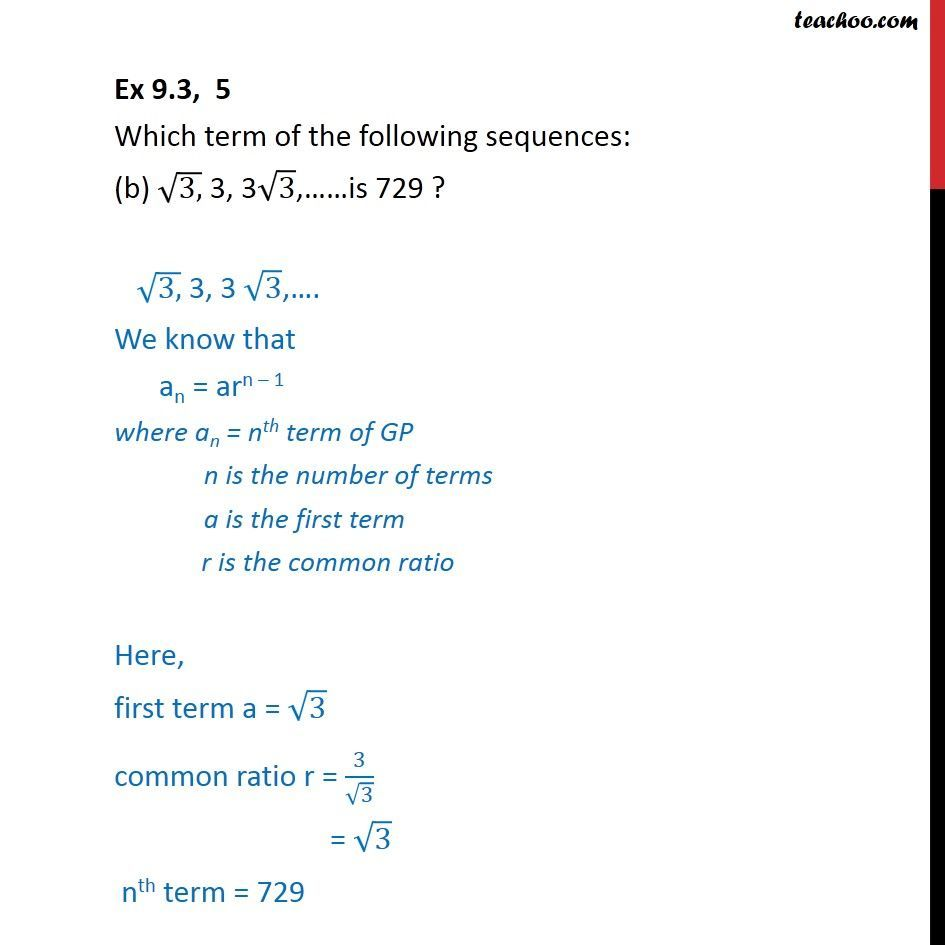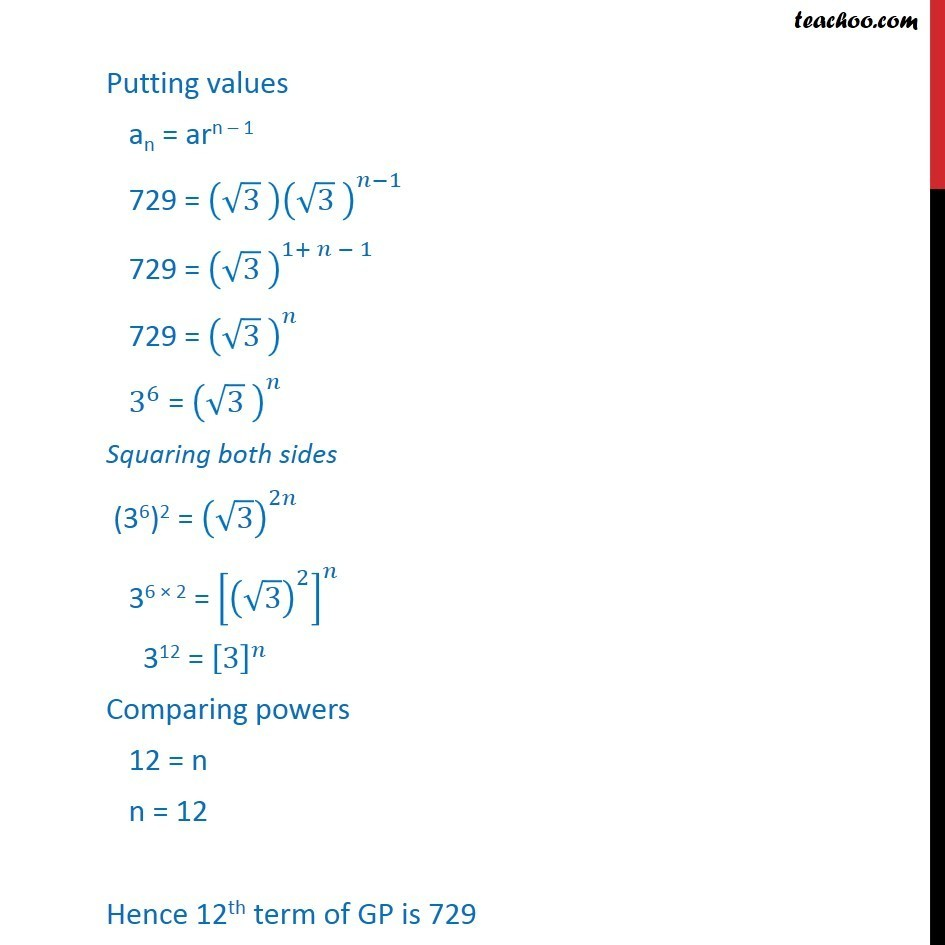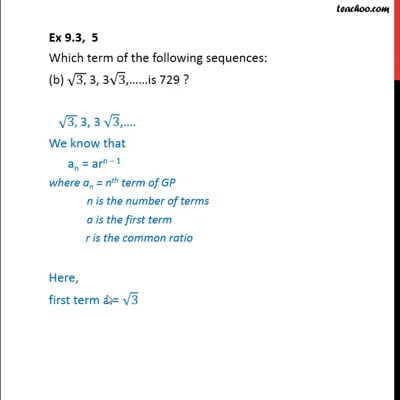Ex 9.3

Chapter 9 Class 11 Sequences and Series
Serial order wiseThis video is only available for Teachoo black users

Maths Crash Course - Live lectures + all videos + Real time Doubt solving!

### Transcript

Ex 9.3, 5 Which term of the following sequences: (b) (3,) 3, 3 3, is 729 ? (3,) 3, 3 3, . We know that an = arn 1 where an = nth term of GP n is the number of terms a is the first term r is the common ratio Here, first term a = 3 common ratio r = 3/ 3 = 3 nth term = 729 Putting values an = arn 1 729 = ( 3 ) ( 3 )^( 1) 729 = ( 3 )^(1+ 1) 729 = ( 3 )^ 3^6 = ( 3 )^ Squaring both sides (36)2 = ( 3)^2 36 2 = [( 3)^2 ]^ 312 = ^ Comparing powers 12 = n n = 12 Hence 12th term of GP is 729